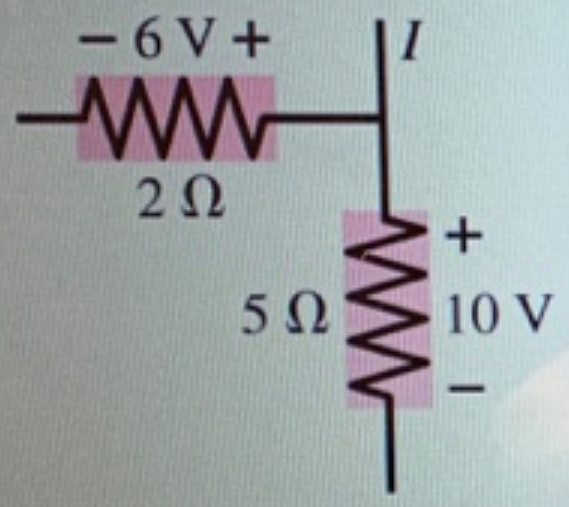# Problem: Part A In (Figure 1), what is the magnitude of the current in the wire above the junction? Express your answer in amperes. Part B Consider the wire above the junction we are looking from the top of the figure. Does charge flow toward or away from the junction? A. toward B. away

###### FREE Expert Solution

A.

From Kirchhoff's junction rule:

$\overline{){{\mathbf{i}}}_{\mathbf{i}\mathbf{n}}{\mathbf{=}}{{\mathbf{i}}}_{\mathbf{o}\mathbf{u}\mathbf{t}}}$

95% (471 ratings)###### Problem Details

Part A In (Figure 1), what is the magnitude of the current in the wire above the junction? Express your answer in amperes.

Part B Consider the wire above the junction we are looking from the top of the figure. Does charge flow toward or away from the junction?

A. toward

B. away